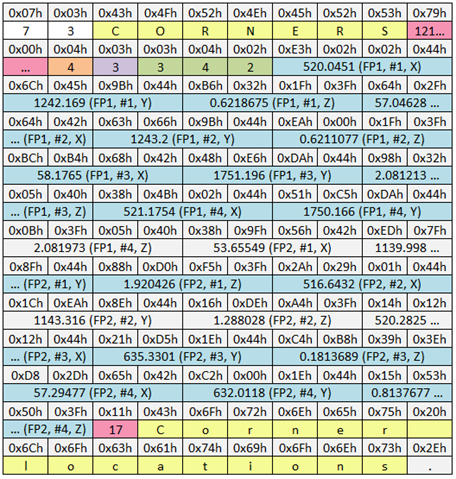﻿ Parameter Arrays
 The Parameter Section > C3D Groups and Parameters

### Parameter Arrays

The parameter format used by arrays is similar to the scalar parameters with the addition of a series of byte values that describe the array dimensions.  For example, the CORNERS parameter in the FORCE_PLATFORM group (which is always a 3, 4, n array where n is the number of plates supported) is stored as follows:Figure 19 - This FORCE_PLATFORM:CORNERS parameter is a (3,4,2) array.

All parameters use the same basic structure so the parameter starts with the length of the parameter name and the group ID that the parameter belongs to, followed by the name.  In this case the parameter name is 7 characters long and the parameter has a GROUP ID of 3 which, in this file is the FORCE_PLATFORM group.  As before, the word following the parameter name effectively points to the start of the next parameter (if any) and then a single byte that defines the storage format used by the parameter (again 4 specifying floating-point values for the parameter).

The following byte is not 0 – indicating that this parameter contains an array – the value stored here indicates the number of array dimensions and should be a value between 1 and 7 (0 here would indicate a scalar parameter).  The following bytes (three in this example) describe the size of each of the arrays enumerated by this byte.

The parameter section of the C3D file follows FORTRAN convention and stores array in column order, thus the FORCE_PLATFORM:CORNERS array, consisting of the X, Y, and Z coordinates of each of the four force plate corners, will look like this for a C3D file with two (P1 & P2) force plates:

P1#1x P1#2x P1#3x P1#4x P2#1x P2#2x P2#3x P2#4x

P1#1y P1#2y P1#3y P1#4y P2#1y P2#2y P2#3y P2#4x

P1#1z P1#2z P1#3z P1#4z P2#1z P2#2z P2#3z P2#4z

This array is stored serially in the C3D parameter in the order P1#1x, P1#1y, P1#1z, P1#2x, P1#2y, P1#2z, P1#3x, P1#3y, P1#3z, P1#4x, P1#4y, P1#4z, P2#1x, P2#1y, P2#1z, P2#2x, P2#2y, P2#2z, P2#3x, P2#3y, P2#3z, P2#4x, P2#4y, P2#4z.

In FORTRAN, and C3D parameter notation, these elements are written as C(1,1,1), C(2,1,1), C(3,1,1), C(1,2,1), C(2,2,1), C(3,2,1), C(1,3,1), C(2,3,1), C(3,3,1), C(1,4,1), C(2,4,1), C(3,4,1), C(1,1,2), C(2,1,2), C(3,1,2), C(1,2,2), C(2,2,2), C(3,2,2), C(1,3,2), C(2,3,2), C(3,3,2), C(1,4,2), C(2,4,2), C(3,4,2), and the array is dimensioned as C(3,4,2).

Software applications reading and processing data in C3D arrays must ensure that the elements in the array are used correctly.  If care if not taken then confusion can arise in the way matrices are processed due to the differences between the default FORTRAN column order of the array and the row based order assumed by C or other C++ based languages.